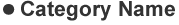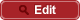• HOME
• RIKEN-QHP
• Observation of $e^+ e^－ \to \gamma \chi_{c1}$ and search for $e^+ e^－ \to \gamma \chi_{c0}, \gamma \chi_{c2}$, and $\gamma \eta_c$ at $\sqrt{s}$ near 10.6 GeV at Belle

##PrePrint No. RIKEN-QHP-398 S. Jia et al., (Belle Collaboration) including M. Takizawa Observation of $e^+ e^－ \to \gamma \chi_{c1}$ and search for $e^+ e^－ \to \gamma \chi_{c0}, \gamma \chi_{c2}$, and $\gamma \eta_c$ at $\sqrt{s}$ near 10.6 GeV at Belle arXiv: 1810.10291  (org) Phys. Rev. D, 092015(R) (2018) 2018.10